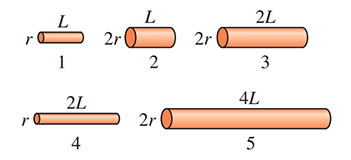# Problem: The wires in (Figure 1) all have the same resistance; the length and radius of each wire are noted. Rank in order, from largest to smallest, the resistivities ρ1 to ρ5 of these wires.

###### FREE Expert Solution

Resistance:

$\overline{){\mathbf{R}}{\mathbf{=}}\frac{\mathbf{\rho }\mathbf{L}}{\mathbf{A}}{\mathbf{=}}\frac{\mathbf{\rho }\mathbf{L}}{\mathbf{\pi }{\mathbf{r}}^{\mathbf{2}}}}$

ρ = R•πr2/L

ρ1 = πR•(r2/L)###### Problem Details

The wires in (Figure 1) all have the same resistance; the length and radius of each wire are noted. Rank in order, from largest to smallest, the resistivities ρ1 to ρ5 of these wires.